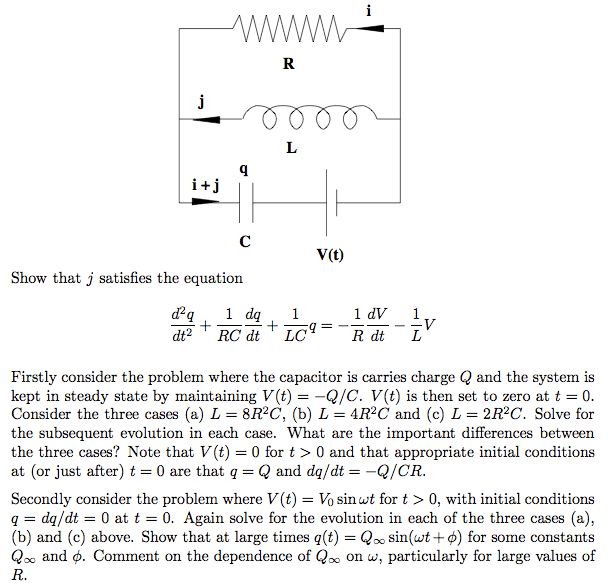# RLC circuit (again)

## Homework Statement## The Attempt at a Solution

Ok, first part, no problem, second part (steady state), solved in another thread. Both are pretty tedious, but doable.

However, I am quite stumped by the third part of this problem (V = V_o sin wt) .

The second part of the problem requires me to use a 2nd order differential equation (y'' + ay' + b = 0), and the solutions of the auxiliary equation can be saved for the third part.

For the third part, I choose a particular integral y = A sin wt + B cos wt. The last part arises essentially because the GS of the third part is y = exponent + exponent + A sin wt + B cos wt, and the first two terms become 0 when t is large. Then I can reexpress A sin wt + B cos wt into the form required.

I have just tried to go about the third part the standard way (differentiating and substituting the particular integral to find A and B, and then substituting again to find the constants in the complementary function), but it is extremely tedious (one page working just to find and simplify A or B) and I hope there is a shorter solution.